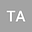Analysis of Two-operator Boundary-Domain Integral Equations for Variable Coefficient BVPs with General Data
•## Abstract

The Dirichlet, Neumann and mixed boundary value problems for the linear second-order scalar elliptic differential equation with variable coefficient in a bounded three-dimensional domain are considered. The PDE right-hand side belongs to $H^{-1}(\Omega)$ or $\widetilde{H}^{-1}(\Omega),$ when neither classical nor canonical co-normal derivatives are well defined. Using the two-operator approach and appropriate parametrix (Levi function) each problem is reduced to different systems of boundary domain integral equations (BDIEs). Equivalence of the BDIEs to the original BVP, BDIE solvability, solution uniqueness/non-uniqueness, and as well as invertibility of the BDIE operators are analysed in appropriate Sobolev (Bessel potential) spaces. It is shown that the BDIE operators for the Neumann BVP are not invertible, and appropriate finite-dimensional perturbations are constructed leading to invertibility of the perturbed operators.
16 Jan 2020Submitted to Mathematical Methods in the Applied Sciences
18 Jan 2020Submission Checks Completed
18 Jan 2020Assigned to Editor
01 Feb 2020Reviewer(s) Assigned
11 Apr 2020Review(s) Completed, Editorial Evaluation Pending
04 Jul 2020Editorial Decision: Revise Minor